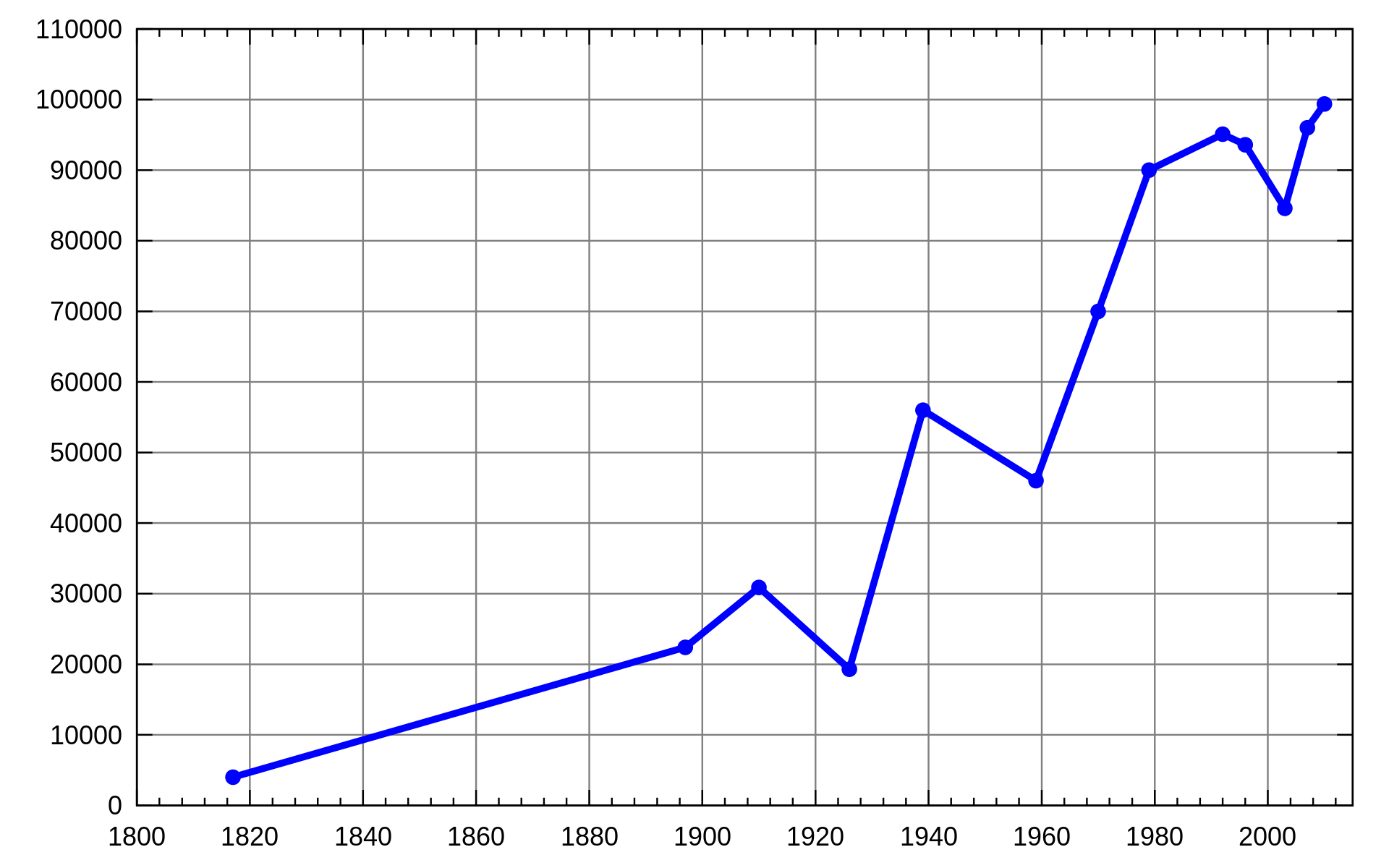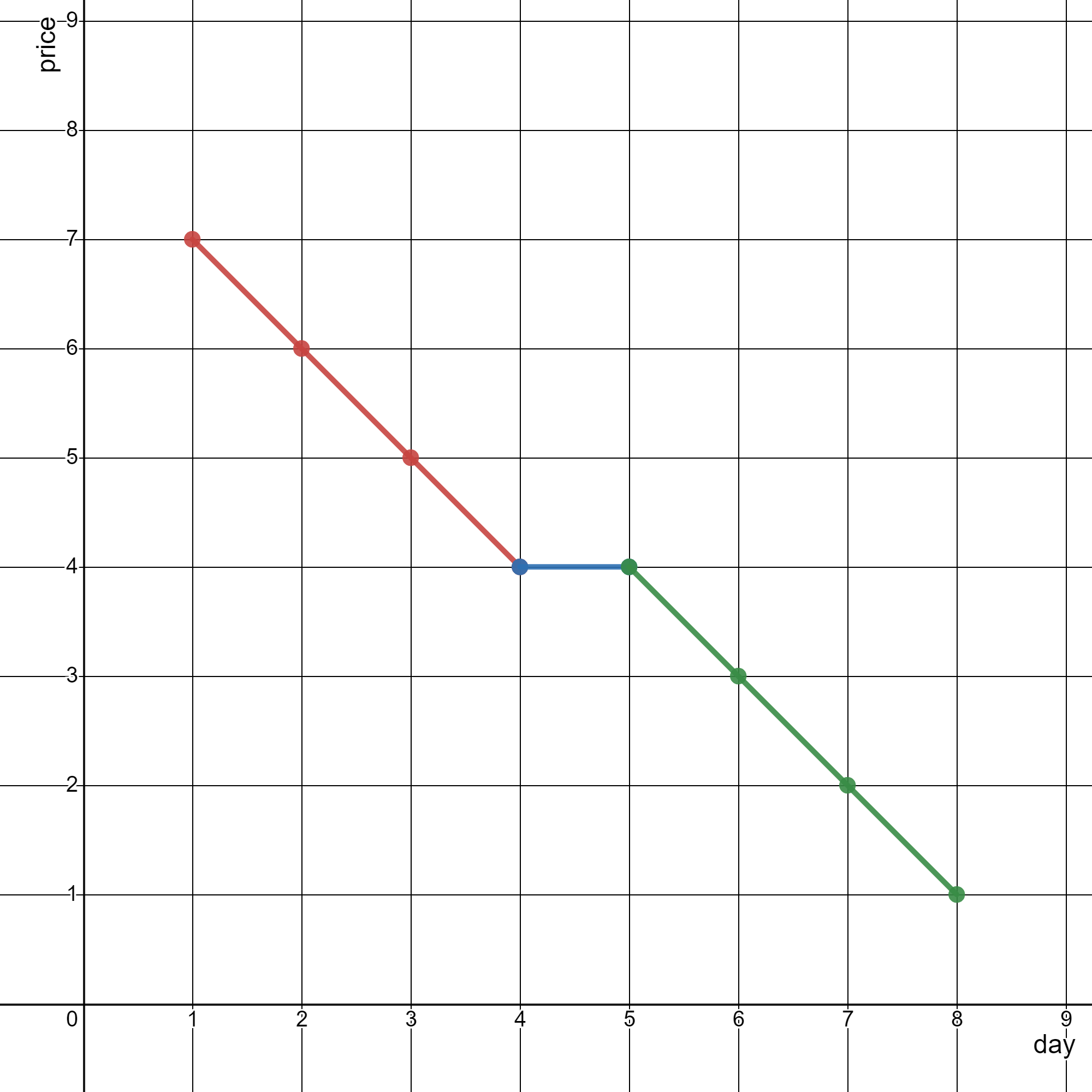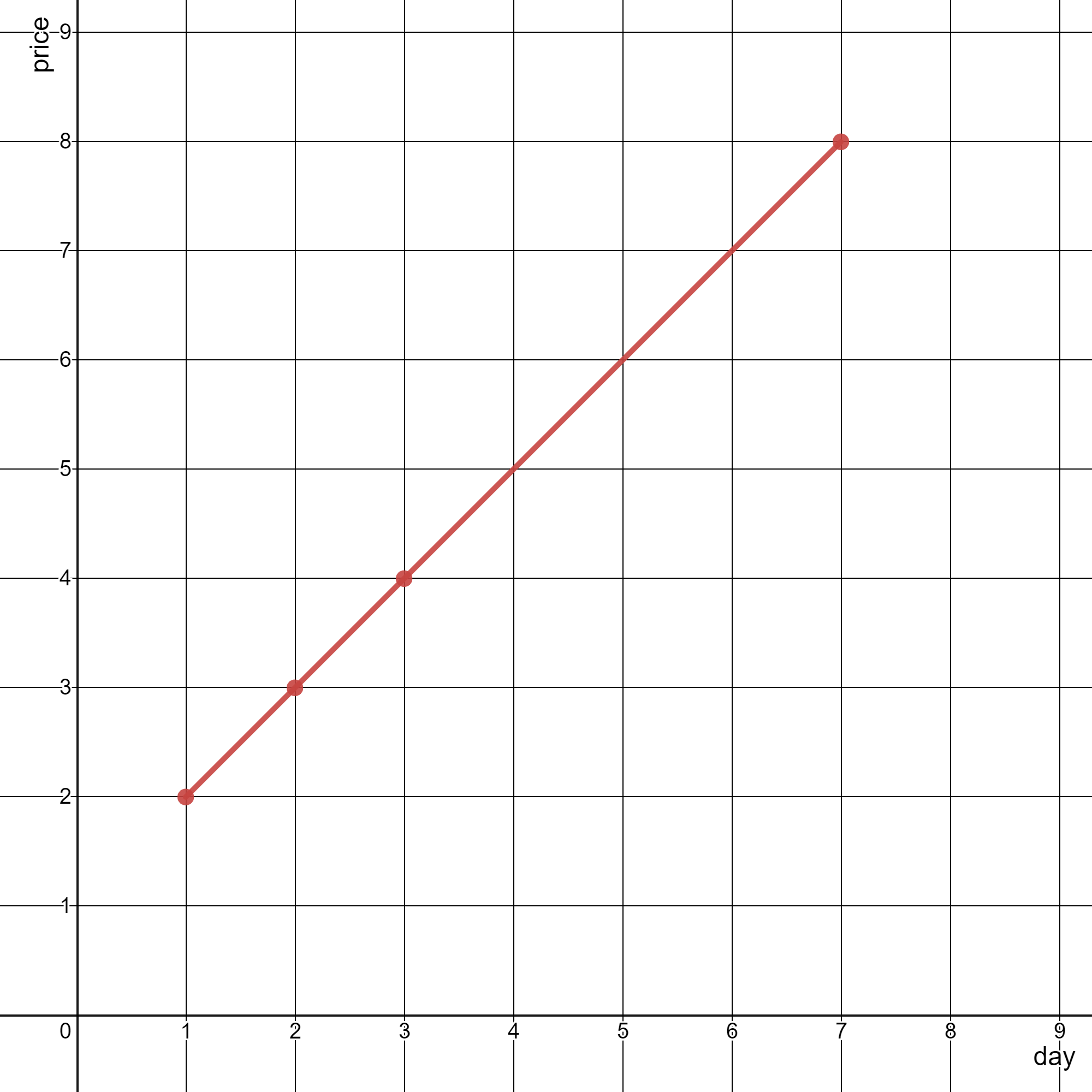# weekly-contest-294

## A

### Statement

``````输入：s = "foobar", letter = "o"

``````

``````输入：s = "jjjj", letter = "k"

• `1 <= s.length <= 100`
• `s` 由小写英文字母组成
• `letter` 是一个小写英文字母

Given a string `s` and a character `letter`, return the percentage of characters in `s` that equal `letter` rounded down to the nearest whole percent.

Example 1:

``````Input: s = "foobar", letter = "o"
Output: 33
Explanation:
The percentage of characters in s that equal the letter 'o' is 2 / 6 * 100% = 33% when rounded down, so we return 33.
``````

Example 2:

``````Input: s = "jjjj", letter = "k"
Output: 0
Explanation:
The percentage of characters in s that equal the letter 'k' is 0%, so we return 0.``````

Constraints:

• `1 <= s.length <= 100`
• `s` consists of lowercase English letters.
• `letter` is a lowercase English letter.

### Solution

``````#include <bits/stdc++.h>
#include <ext/pb_ds/assoc_container.hpp>
#include <ext/pb_ds/tree_policy.hpp>

#define endl "\n"
#define fi first
#define se second
#define all(x) begin(x), end(x)
#define rall rbegin(a), rend(a)
#define bitcnt(x) (__builtin_popcountll(x))
#define complete_unique(a) a.erase(unique(begin(a), end(a)), end(a))
#define mst(x, a) memset(x, a, sizeof(x))
#define MP make_pair

using ll = long long;
using ull = unsigned long long;
using db = double;
using ld = long double;
using VLL = std::vector<ll>;
using VI = std::vector<int>;
using PII = std::pair<int, int>;
using PLL = std::pair<ll, ll>;

using namespace __gnu_pbds;
using namespace std;
template <typename T>
using ordered_set = tree<T, null_type, less<T>, rb_tree_tag, tree_order_statistics_node_update>;

template <typename T, typename S>
inline bool chmax(T &a, const S &b) {
return a < b ? a = b, 1 : 0;
}

template <typename T, typename S>
inline bool chmin(T &a, const S &b) {
return a > b ? a = b, 1 : 0;
}

#ifdef LOCAL
#include <debug.hpp>
#else
#define dbg(...)
#endif

class Solution {
public:
int percentageLetter(string s, char letter) {
int tot = s.length();
int cur = 0;

for (const char &c : s) {
if (c == letter) {
++cur;
}
}

return cur * 100 / tot;
}
};
#ifdef LOCAL

int main() {
return 0;
}

#endif
``````

## B

### Statement

``````输入：capacity = [2,3,4,5], rocks = [1,2,4,4], additionalRocks = 2

1 块石头放入背包 0 ，1 块石头放入背包 1 。

``````

``````输入：capacity = [10,2,2], rocks = [2,2,0], additionalRocks = 100

8 块石头放入背包 0 ，2 块石头放入背包 2 。

``````

• `n == capacity.length == rocks.length`
• `1 <= n <= 5 * 104`
• `1 <= capacity[i] <= 109`
• `0 <= rocks[i] <= capacity[i]`
• `1 <= additionalRocks <= 109`

You have `n` bags numbered from `0` to `n - 1`. You are given two 0-indexed integer arrays `capacity` and `rocks`. The `ith` bag can hold a maximum of `capacity[i]` rocks and currently contains `rocks[i]` rocks. You are also given an integer `additionalRocks`, the number of additional rocks you can place in any of the bags.

Return the maximum number of bags that could have full capacity after placing the additional rocks in some bags.

Example 1:

``````Input: capacity = [2,3,4,5], rocks = [1,2,4,4], additionalRocks = 2
Output: 3
Explanation:
Place 1 rock in bag 0 and 1 rock in bag 1.
The number of rocks in each bag are now [2,3,4,4].
Bags 0, 1, and 2 have full capacity.
There are 3 bags at full capacity, so we return 3.
It can be shown that it is not possible to have more than 3 bags at full capacity.
Note that there may be other ways of placing the rocks that result in an answer of 3.
``````

Example 2:

``````Input: capacity = [10,2,2], rocks = [2,2,0], additionalRocks = 100
Output: 3
Explanation:
Place 8 rocks in bag 0 and 2 rocks in bag 2.
The number of rocks in each bag are now [10,2,2].
Bags 0, 1, and 2 have full capacity.
There are 3 bags at full capacity, so we return 3.
It can be shown that it is not possible to have more than 3 bags at full capacity.
Note that we did not use all of the additional rocks.
``````

Constraints:

• `n == capacity.length == rocks.length`
• `1 <= n <= 5 * 104`
• `1 <= capacity[i] <= 109`
• `0 <= rocks[i] <= capacity[i]`
• `1 <= additionalRocks <= 109`

### Solution

``````#include <bits/stdc++.h>
#include <ext/pb_ds/assoc_container.hpp>
#include <ext/pb_ds/tree_policy.hpp>

#define endl "\n"
#define fi first
#define se second
#define all(x) begin(x), end(x)
#define rall rbegin(a), rend(a)
#define bitcnt(x) (__builtin_popcountll(x))
#define complete_unique(a) a.erase(unique(begin(a), end(a)), end(a))
#define mst(x, a) memset(x, a, sizeof(x))
#define MP make_pair

using ll = long long;
using ull = unsigned long long;
using db = double;
using ld = long double;
using VLL = std::vector<ll>;
using VI = std::vector<int>;
using PII = std::pair<int, int>;
using PLL = std::pair<ll, ll>;

using namespace __gnu_pbds;
using namespace std;
template <typename T>
using ordered_set = tree<T, null_type, less<T>, rb_tree_tag, tree_order_statistics_node_update>;

template <typename T, typename S>
inline bool chmax(T &a, const S &b) {
return a < b ? a = b, 1 : 0;
}

template <typename T, typename S>
inline bool chmin(T &a, const S &b) {
return a > b ? a = b, 1 : 0;
}

#ifdef LOCAL
#include <debug.hpp>
#else
#define dbg(...)
#endif

class Solution {
public:
int maximumBags(vector<int> &capacity, vector<int> &rocks, int additionalRocks) {
auto need = vector<int>();
int n = capacity.size();

for (int i = 0; i < n; i++) {
need.push_back(capacity[i] - rocks[i]);
}

sort(need.begin(), need.end());
reverse(need.begin(), need.end());

int res = 0;

while (!need.empty()) {
int cur = need.back();
need.pop_back();

break;
}

++res;
}

return res;
}
};

#ifdef LOCAL

int main() {
return 0;
}

#endif
``````

## C

### Statement``````输入：stockPrices = [[1,7],[2,6],[3,5],[4,4],[5,4],[6,3],[7,2],[8,1]]

- 线段 1 （红色）从 (1,7) 到 (4,4) ，经过 (1,7) ，(2,6) ，(3,5) 和 (4,4) 。
- 线段 2 （蓝色）从 (4,4) 到 (5,4) 。
- 线段 3 （绿色）从 (5,4) 到 (8,1) ，经过 (5,4) ，(6,3) ，(7,2) 和 (8,1) 。

````````````输入：stockPrices = [[3,4],[1,2],[7,8],[2,3]]

``````

• `1 <= stockPrices.length <= 105`
• `stockPrices[i].length == 2`
• `1 <= dayi, pricei <= 109`
• 所有 `dayi` 互不相同 。

You are given a 2D integer array `stockPrices` where `stockPrices[i] = [dayi, pricei]` indicates the price of the stock on day `dayi` is `pricei`. A line chart is created from the array by plotting the points on an XY plane with the X-axis representing the day and the Y-axis representing the price and connecting adjacent points. One such example is shown below:Return the minimum number of lines needed to represent the line chart.

Example 1:``````Input: stockPrices = [[1,7],[2,6],[3,5],[4,4],[5,4],[6,3],[7,2],[8,1]]
Output: 3
Explanation:
The diagram above represents the input, with the X-axis representing the day and Y-axis representing the price.
The following 3 lines can be drawn to represent the line chart:
- Line 1 (in red) from (1,7) to (4,4) passing through (1,7), (2,6), (3,5), and (4,4).
- Line 2 (in blue) from (4,4) to (5,4).
- Line 3 (in green) from (5,4) to (8,1) passing through (5,4), (6,3), (7,2), and (8,1).
It can be shown that it is not possible to represent the line chart using less than 3 lines.
``````

Example 2:``````Input: stockPrices = [[3,4],[1,2],[7,8],[2,3]]
Output: 1
Explanation:
As shown in the diagram above, the line chart can be represented with a single line.
``````

Constraints:

• `1 <= stockPrices.length <= 105`
• `stockPrices[i].length == 2`
• `1 <= dayi, pricei <= 109`
• All `dayi` are distinct.

### Solution

``````#include <bits/stdc++.h>
#include <ext/pb_ds/assoc_container.hpp>
#include <ext/pb_ds/tree_policy.hpp>

#define endl "\n"
#define fi first
#define se second
#define all(x) begin(x), end(x)
#define rall rbegin(a), rend(a)
#define bitcnt(x) (__builtin_popcountll(x))
#define complete_unique(a) a.erase(unique(begin(a), end(a)), end(a))
#define mst(x, a) memset(x, a, sizeof(x))
#define MP make_pair

using ll = long long;
using ull = unsigned long long;
using db = double;
using ld = long double;
using VLL = std::vector<ll>;
using VI = std::vector<int>;
using PII = std::pair<int, int>;
using PLL = std::pair<ll, ll>;

using namespace __gnu_pbds;
using namespace std;
template <typename T>
using ordered_set = tree<T, null_type, less<T>, rb_tree_tag, tree_order_statistics_node_update>;

template <typename T, typename S>
inline bool chmax(T &a, const S &b) {
return a < b ? a = b, 1 : 0;
}

template <typename T, typename S>
inline bool chmin(T &a, const S &b) {
return a > b ? a = b, 1 : 0;
}

#ifdef LOCAL
#include <debug.hpp>
#else
#define dbg(...)
#endif

class Solution {
public:
int minimumLines(vector<vector<int>> &s) {
int n = s.size();

if (n == 1) {
return 0;
}

if (n <= 2) {
return 1;
}

sort(s.begin(), s.end(), [&](const vector<int> &a, const vector<int> &b) {
return a < b;
});

const auto ok = [](vector<int> a, vector<int> b, vector<int> c) {
ll aa = 1ll * (b - a) * (c - a);
ll bb = 1ll * (b - a) * (c - a);

return aa == bb;
};

int res = 1;
int l = 0;
for (int i = 2; i < n; i++) {
if (ok(s[l], s[l + 1], s[i])) {
continue;
}

l = i - 1;
++res;
}

return res;
}
};

#ifdef LOCAL

int main() {
return 0;
}

#endif
``````

## D

### Statement

• Difficulty: Hard
• Tag: `栈` `数组` `前缀和` `单调栈`

• 巫师中 最弱 的能力值。
• 组中所有巫师的个人力量值 之和 。

``````输入：strength = [1,3,1,2]

- [1,3,1,2] 中  ，总力量值为 min() * sum() = 1 * 1 = 1
- [1,3,1,2] 中  ，总力量值为 min() * sum() = 3 * 3 = 9
- [1,3,1,2] 中  ，总力量值为 min() * sum() = 1 * 1 = 1
- [1,3,1,2] 中  ，总力量值为 min() * sum() = 2 * 2 = 4
- [1,3,1,2] 中 [1,3] ，总力量值为 min([1,3]) * sum([1,3]) = 1 * 4 = 4
- [1,3,1,2] 中 [3,1] ，总力量值为 min([3,1]) * sum([3,1]) = 1 * 4 = 4
- [1,3,1,2] 中 [1,2] ，总力量值为 min([1,2]) * sum([1,2]) = 1 * 3 = 3
- [1,3,1,2] 中 [1,3,1] ，总力量值为 min([1,3,1]) * sum([1,3,1]) = 1 * 5 = 5
- [1,3,1,2] 中 [3,1,2] ，总力量值为 min([3,1,2]) * sum([3,1,2]) = 1 * 6 = 6
- [1,3,1,2] 中 [1,3,1,2] ，总力量值为 min([1,3,1,2]) * sum([1,3,1,2]) = 1 * 7 = 7

``````

``````输入：strength = [5,4,6]

- [5,4,6] 中  ，总力量值为 min() * sum() = 5 * 5 = 25
- [5,4,6] 中  ，总力量值为 min() * sum() = 4 * 4 = 16
- [5,4,6] 中  ，总力量值为 min() * sum() = 6 * 6 = 36
- [5,4,6] 中 [5,4] ，总力量值为 min([5,4]) * sum([5,4]) = 4 * 9 = 36
- [5,4,6] 中 [4,6] ，总力量值为 min([4,6]) * sum([4,6]) = 4 * 10 = 40
- [5,4,6] 中 [5,4,6] ，总力量值为 min([5,4,6]) * sum([5,4,6]) = 4 * 15 = 60

``````

• `1 <= strength.length <= 105`
• `1 <= strength[i] <= 109`

As the ruler of a kingdom, you have an army of wizards at your command.

You are given a 0-indexed integer array `strength`, where `strength[i]` denotes the strength of the `ith` wizard. For a contiguous group of wizards (i.e. the wizards' strengths form a subarray of `strength`), the total strength is defined as the product of the following two values:

• The strength of the weakest wizard in the group.
• The total of all the individual strengths of the wizards in the group.

Return the sum of the total strengths of all contiguous groups of wizards. Since the answer may be very large, return it modulo `109 + 7`.

A subarray is a contiguous non-empty sequence of elements within an array.

Example 1:

``````Input: strength = [1,3,1,2]
Output: 44
Explanation: The following are all the contiguous groups of wizards:
-  from [1,3,1,2] has a total strength of min() * sum() = 1 * 1 = 1
-  from [1,3,1,2] has a total strength of min() * sum() = 3 * 3 = 9
-  from [1,3,1,2] has a total strength of min() * sum() = 1 * 1 = 1
-  from [1,3,1,2] has a total strength of min() * sum() = 2 * 2 = 4
- [1,3] from [1,3,1,2] has a total strength of min([1,3]) * sum([1,3]) = 1 * 4 = 4
- [3,1] from [1,3,1,2] has a total strength of min([3,1]) * sum([3,1]) = 1 * 4 = 4
- [1,2] from [1,3,1,2] has a total strength of min([1,2]) * sum([1,2]) = 1 * 3 = 3
- [1,3,1] from [1,3,1,2] has a total strength of min([1,3,1]) * sum([1,3,1]) = 1 * 5 = 5
- [3,1,2] from [1,3,1,2] has a total strength of min([3,1,2]) * sum([3,1,2]) = 1 * 6 = 6
- [1,3,1,2] from [1,3,1,2] has a total strength of min([1,3,1,2]) * sum([1,3,1,2]) = 1 * 7 = 7
The sum of all the total strengths is 1 + 9 + 1 + 4 + 4 + 4 + 3 + 5 + 6 + 7 = 44.
``````

Example 2:

``````Input: strength = [5,4,6]
Output: 213
Explanation: The following are all the contiguous groups of wizards:
-  from [5,4,6] has a total strength of min() * sum() = 5 * 5 = 25
-  from [5,4,6] has a total strength of min() * sum() = 4 * 4 = 16
-  from [5,4,6] has a total strength of min() * sum() = 6 * 6 = 36
- [5,4] from [5,4,6] has a total strength of min([5,4]) * sum([5,4]) = 4 * 9 = 36
- [4,6] from [5,4,6] has a total strength of min([4,6]) * sum([4,6]) = 4 * 10 = 40
- [5,4,6] from [5,4,6] has a total strength of min([5,4,6]) * sum([5,4,6]) = 4 * 15 = 60
The sum of all the total strengths is 25 + 16 + 36 + 36 + 40 + 60 = 213.
``````

Constraints:

• `1 <= strength.length <= 105`
• `1 <= strength[i] <= 109`

### Solution

``````#include <bits/stdc++.h>
#include <ext/pb_ds/assoc_container.hpp>
#include <ext/pb_ds/tree_policy.hpp>
#include <vector>

#define endl "\n"
#define fi first
#define se second
#define all(x) begin(x), end(x)
#define rall rbegin(a), rend(a)
#define bitcnt(x) (__builtin_popcountll(x))
#define complete_unique(a) a.erase(unique(begin(a), end(a)), end(a))
#define mst(x, a) memset(x, a, sizeof(x))
#define MP make_pair

using ll = long long;
using ull = unsigned long long;
using db = double;
using ld = long double;
using VLL = std::vector<ll>;
using VI = std::vector<int>;
using PII = std::pair<int, int>;
using PLL = std::pair<ll, ll>;

using namespace __gnu_pbds;
using namespace std;
template <typename T>
using ordered_set = tree<T, null_type, less<T>, rb_tree_tag, tree_order_statistics_node_update>;

template <typename T, typename S>
inline bool chmax(T &a, const S &b) {
return a < b ? a = b, 1 : 0;
}

template <typename T, typename S>
inline bool chmin(T &a, const S &b) {
return a > b ? a = b, 1 : 0;
}

#ifdef LOCAL
#include <debug.hpp>
#else
#define dbg(...)
#endif

const int mod = 1e9 + 7;

struct node {
int x, l, r;

ll GetSum(const vector<ll> &suffix_sum) {
ll ans = 0;

ans += 1ll * x * (suffix_sum[l] - suffix_sum[r + 1] + mod) % mod;
ans %= mod;

return ans;
}

ll GetBaseSum() {
return 1ll * x * (r - l + 1);
}
};

class Solution {
public:
int totalStrength(vector<int> &a) {
int n = (int)a.size();
vector<vector<ll>> suffix_sum(2, vector<ll>(n + 5, 0));

for (int i = n; i >= 1; i--) {
suffix_sum[i] = (suffix_sum[i + 1] + a[i - 1]) % mod;
}

for (int i = n; i >= 1; i--) {
suffix_sum[i] = (suffix_sum[i + 1] + suffix_sum[i]) % mod;
}

vector<node> v;

ll sum = 0;
ll base_sum = 0;
ll res = 0;

for (int i = 0; i < n; i++) {
auto t = node();
t.x = a[i];
t.l = i + 1;
t.r = i + 1;

while (!v.empty()) {
if (v.back().x < t.x) {
break;
}

sum = (sum - v.back().GetSum(suffix_sum) + mod) % mod;
base_sum = (base_sum - v.back().GetBaseSum() + mod) % mod;
t.l = v.back().l;
v.pop_back();
}

v.push_back(t);
sum = (sum + t.GetSum(suffix_sum)) % mod;
base_sum = (base_sum + t.GetBaseSum()) % mod;
res = (res + sum) % mod;
res -= 1ll * base_sum * suffix_sum[i + 2] % mod;
res += mod;
res %= mod;
}

return int(res);
}
};

#ifdef LOCAL

int main() {
return 0;
}

#endif
``````